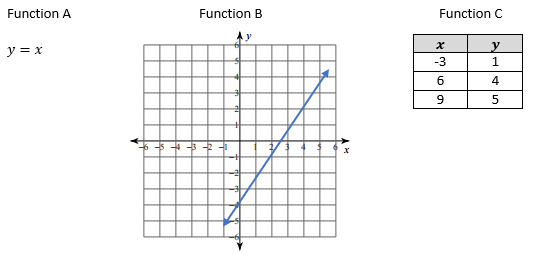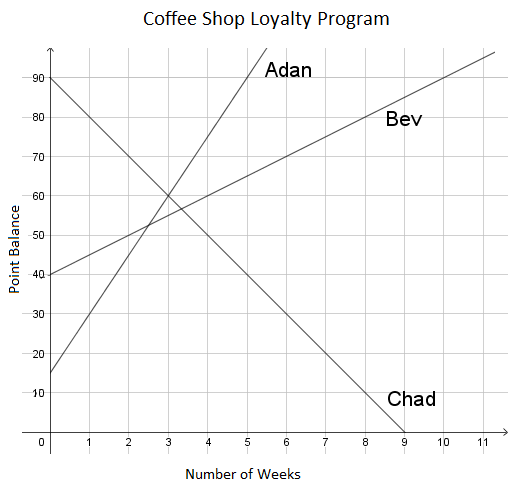# Linear Relationships

## Objective

Compare linear functions represented in different ways.

## Common Core Standards

### Core Standards

?

• 8.F.A.2 — Compare properties of two functions each represented in a different way (algebraically, graphically, numerically in tables, or by verbal descriptions). For example, given a linear function represented by a table of values and a linear function represented by an algebraic expression, determine which function has the greater rate of change.

• 8.F.B.4 — Construct a function to model a linear relationship between two quantities. Determine the rate of change and initial value of the function from a description of a relationship or from two (x, y) values, including reading these from a table or from a graph. Interpret the rate of change and initial value of a linear function in terms of the situation it models, and in terms of its graph or a table of values.

## Criteria for Success

?

1. Identify slope and $y$-intercept of lines represented as graphs, tables, and equations.
2. Compare the slopes and $y$-intercepts of linear functions.

## Tips for Teachers

?

In Lessons 9 and 10 of Unit 4, students compared functions by looking at the rates of change and initial values of those functions. This lesson revisits some of those concepts, but looks specifically at linear functions and describes the graphs of these functions by the slope and $y$-intercept of the line.

#### Fishtank Plus

• Problem Set
• Student Handout Editor
• Vocabulary Package

## Anchor Problems

?

### Problem 1

Three linear functions are represented below.1. Order the functions by the slope of the line that represents each function, from least to greatest.
2. Order the functions by the $y$-intercept of the line that represents each function, from least to greatest.

### Problem 2

Three friends participate in the loyalty program at a local coffee shop. A participant in the program earns points for each visit that can be used to get drinks and food for free. The graph below shows the point balances of the three friends over several weeks.1. Describe what each line tells you about the friends’ point balance. What can you tell about how they earn and use their points?
2. Who has the most points at the start?
3. Write an equation for the point balance for each friend.
4. A fourth friend has a point balance represented by the equation ${y=12x+50}$. Does this friend earn points at a faster or slower rate than Adan?

### Problem 3

Line L goes through the points (4, 9) and (-4, -7). Line M has a $y-$intercept that is greater than line L.

What could be the equation for line M?

1.   ${y={1\over2}x+8}$
2.   ${y={2\over3}x-8}$
3.   ${y=2x}$
4.   ${y=3x-5}$

## Problem Set

?The following resources include problems and activities aligned to the objective of the lesson that can be used to create your own problem set.

• Include multiple-choice problems where students are presented with two linear functions and determine true statements that compare slope and/or y-intercepts of the lines.
• EngageNY Mathematics Grade 8 Mathematics > Module 5 > Topic A > Lesson 7Revisit the Problem Set; this was included as a resource in Unit 4 Lesson 9 but may be worthwhile returning to in light of the new concepts learned in this unit.
• MARS Formative Assessment Lesson for Grade 8 Comparing Lines and Linear EquationsGreat activity where students are required to pay close attention to how graphs and equations represent different rates.

?

Linear function $K$ is represented by the equation ${y={1\over2}x-2}$.

Draw the graph of a line that has a greater slope than function $K$ and the same $y$-intercept as function $K$.?